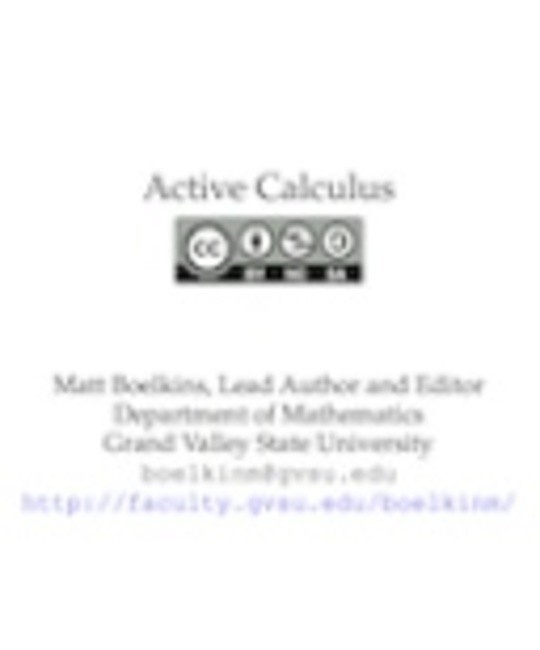Free

# Active Calculus

By Matt Boelkins
Free
Book Description

Several fundamental ideas in calculus are more than 2000 years old. As a formal subdiscipline of mathematics, calculus was first introduced and developed in the late 1600s, with key independent contributions from Sir Isaac Newton and Gottfried Wilhelm Leibniz. Mathematicians agree that the subject has been understood rigorously since the work of Augustin Louis Cauchy and Karl Weierstrass in the mid 1800s when the field of modern analysis was developed, in part to make sense of the infinitely small quantities on which calculus rests. Hence, as a body of knowledge calculus has been completely understood by experts for at least 150 years. The discipline is one of our great human intellectual achievements: among many spectacular ideas, calculus models how objects fall under the forces of gravity and wind resistance, explains how to compute areas and volumes of interesting shapes, enables us to work rigorously with infinitely small and infinitely large quantities, and connects the varying rates at which quantities change to the total change in the quantities themselves.

While each author of a calculus textbook certainly offers her own creative perspective on the subject, it is hardly the case that many of the ideas she presents are new. Indeed, the mathematics community broadly agrees on what the main ideas of calculus are, as well as their justification and their importance; the core parts of nearly all calculus textbooks are very similar. As such, it is our opinion that in the 21st century – an age where the internet permits seamless and immediate transmission of information – no one should be required to purchase a calculus text to read, to use for a class, or to find a coherent collection of problems to solve. Calculus belongs to humankind, not any individual author or publishing company. Thus, the main purpose of this work is to present a new calculus text that is free. In addition, instructors who are looking for a calculus text should have the opportunity to download the source files and make modifications that they see fit; thus this text is open-source. Since August 2013, Active Calculus has been endorsed by the American Institute of Mathematics and its Open Textbook Initiative.

• Preface
• Understanding the Derivative
• How do we measure velocity?
• The notion of limit
• The derivative of a function at a point
• The derivative function
• Interpreting, estimating, and using the derivative
• The second derivative
• Limits, Continuity, and Differentiability
• The Tangent Line Approximation
• Computing Derivatives
• Elementary derivative rules
• The sine and cosine functions
• The product and quotient rules
• Derivatives of other trigonometric functions
• The chain rule
• Derivatives of Inverse Functions
• Derivatives of Functions Given Implicitly
• Using Derivatives to Evaluate Limits
• Using Derivatives
• Using derivatives to identify extreme values of a function
• Using derivatives to describe families of functions
• Global Optimization
• Applied Optimization
• Related Rates
• The Definite Integral
• Determining distance traveled from velocity
• Riemann Sums
• The Definite Integral
• The Fundamental Theorem of Calculus
• Finding Antiderivatives and Evaluating Integrals
• Constructing Accurate Graphs of Antiderivatives
• The Second Fundamental Theorem of Calculus
• Integration by Substitution
• Integration by Parts
• Other Options for Finding Algebraic Antiderivatives
• Numerical Integration
• Using Definite Integrals
• Using Definite Integrals to Find Area and Length
• Using Definite Integrals to Find Volume
• Density, Mass, and Center of Mass
• Physics Applications: Work, Force, and Pressure
• Improper Integrals
• Differential Equations
• An Introduction to Differential Equations
• Qualitative behavior of solutions to differential equations
• Euler's method
• Separable differential equations
• Modeling with differential equations
• Population Growth and the Logistic Equation
• Sequences and Series
• Sequences
• Geometric Series
• Series of Real Numbers
• Alternating Series
• Taylor Polynomials and Taylor Series
• Power Series
• A Short Table of Integrals
No comment for this book yet, be the first to comment
Also Available On
Categories
Curated Lists
• #### Free Machine Learning Books

11 Books

Pattern Recognition and Machine Learning (Information Science and Statistics)
by Christopher M. Bishop
Data mining
by I. H. Witten
The Elements of Statistical Learning: Data Mining, Inference, and Prediction
by Various
See more...
• #### Free Chemistry Textbooks

9 Books

CK-12 Chemistry
by Various
Concept Development Studies in Chemistry
by John Hutchinson
An Introduction to Chemistry - Atoms First
by Mark Bishop
See more...
• #### Free Mathematics Textbooks

21 Books

Microsoft Word - How to Use Advanced Algebra II.doc
by Jonathan Emmons
Advanced Algebra II: Activities and Homework
by Kenny Felder
de2de
by
See more...
• #### Free Children Books

38 Books

The Sun Who Lost His Way
by
Tania is a Detective
by Kanika G
Firenze_s-Light
by
See more...
• #### Free Java Books

10 Books

Java 3D Programming
by Daniel Selman
The Java EE 6 Tutorial
by Oracle Corporation
JavaKid811
by
See more...
• #### Sts Peter And Paul Preparatory School eBook List

8 Books

Jamaica Primary Social Studies 2nd Edition Student's Book 4
by Eulie Mantock, Trineta Fendall, Clare Eastland